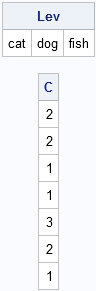A colleague asked, "How can I enumerate the levels of a categorical classification variable in SAS/IML software?" The variable was a character variable with n observations, but he wanted the following:

1. A "look-up table" that contains the k (unique) levels of the variable.
2. A vector with n elements that contains the values 1, 2, ..., k.

### Factors in the R Language

My colleague had previously used the R language, and wanted to duplicate the concept of an R "factor." For example, the following R statements define a character vector, pets, and then use the as.factor function to obtain a factor that has the values 1, 2, and 3 that correspond to the character values "cat," "dog," and "fish":

```# R statements pets <- c("dog","dog","cat","cat","fish","dog","cat") f <- as.factor(pets) str(f) # print structure of f```
```  Factor w/ 3 levels "cat","dog","fish": 2 2 1 1 3 2 1
```

The vector, pets, has three unique levels: "cat","dog", and "fish". The vector {2 2 1 1 3 2 1} specifies that the elements in terms of the look-up table: the first two elements are "dog" (level 2), the third element is "cat" (level 1), and so forth.

### Creating a "Factor" in the SAS/IML Language

Creating a factor is yet another application of the UNIQUE/LOC technique, which is described on p. 69 of my book, Statistical Programming with SAS/IML Software.

The following statements define a SAS/IML module named AsFactor. The third argument is the input argument, x. Upon return, the first argument contains the unique sorted levels of x, whereas the second argument contains integers that correspond to each level of x:

```proc iml; /** AsFactor takes a categorical variable x and returns two matrices: Levels = a row vector that contains the k unique sorted values of x Codes = a matrix that is the same dimensions as x and contains the values 1, 2, ..., k. **/ start AsFactor(Levels, Codes, x); Levels = unique(x); Codes = j(nrow(x),ncol(x)); do i = 1 to ncol(Levels); idx = loc(x=Levels[i]); Codes[idx] = i; end; finish;```

Notice that the output argument, Codes, has the same dimensions as the input argument, x. The following statements call the AsFactor module and print the output values:

```pets = {"dog","dog","cat","cat","fish","dog","cat"}; run AsFactor(Lev, C, pets); print Lev, C;```I'll mention that the AsFactor module is written so that it also works for numeric vectors. For example, the following statements find the unique levels of the numerical vector, Grades:

```grades = {90,90,80,80,100,90,80}; run AsFactor(Lev, C, grades);```

Of course, if you want only the unique levels of a variable in a SAS data set, you can the TABLES statement in the FREQ procedure.

Share